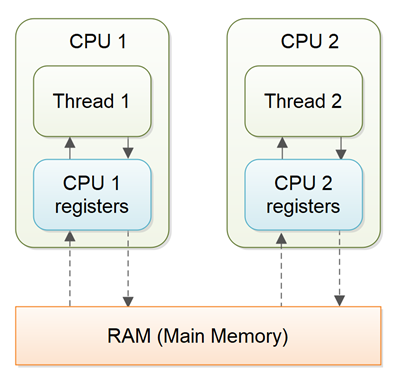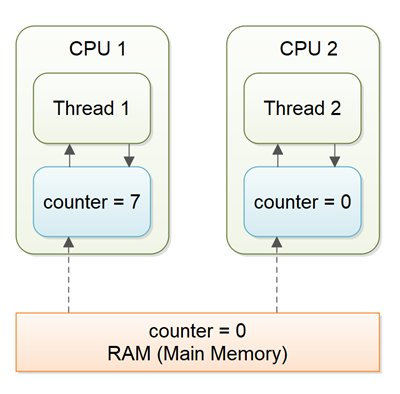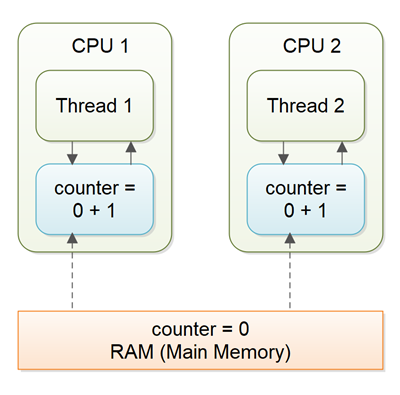Java关键字`volatile`用于将一个Java变量标记为在主内中存储，更准确的解释为：每次读取一个`volatile`变量时将从电脑的主内存中读取而不是从CPU缓存中读取，每次对一个`volatile`变量进行写操作时，将会写入到主内存中而不是写入到CPU缓存中。

## Volatile变量可见性保证

Java中的`volatile`关键字确保了 `volatile`变量的修改在多线程中是可见的。这听起来有些抽象，接下来我将详细说明。``````1 2 3 `````` ``````public class SharedObject { public int counter = 0; } ````````````1 2 3 `````` ``````public class SharedObject { public volatile int counter = 0; } ``````

## Volatile先行发生原则

`Java5`之后`volatile`关键字不仅能用于确保变量从主内存中读取和写入，事实上`volatile`关键字还有如下作用：

• 如果线程A写入了一个`volatile`变量然后线程B读取了这个相同的`volatile`变量，那么所有在线程A写之前对其可见的变量，在线程B读取这个 `volatile`之后也会对其可见。
• `volatile`变量的读写指令不能被JVM重排序（出于性能的考虑，JVM可能会对指令重排序如果JVM检测到指令排序不会对程序运行产生变化）。前后的指令可以重排序，但是`volatile`变量的读和写不能与这些重排序指令混在一起。任何跟随在`volatile`变量读写之后的指令都会确保只有在变量的读写操作之后才能执行。

 ``````1 2 3 4 5 6 7 `````` ``````Thread A: sharedObject.nonVolatile = 123; sharedObject.counter = sharedObject.counter + 1; Thread B: int counter = sharedObject.counter; int nonVolatile = sharedObject.nonVolatile; ``````

 `````` 1 2 3 4 5 6 7 8 9 10 11 12 13 14 15 16 17 18 19 20 21 22 `````` ``````public class Exchanger { private Object object = null; private volatile hasNewObject = false; public void put(Object newObject) { while(hasNewObject) { //等待，不覆盖已经存在的新对象 } object = newObject; hasNewObject = true; //volatile写入 } public Object take(){ while(!hasNewObject){ //volatile读取 //等待，不获取旧的对象（或null对象） } Object obj = object; hasNewObject = false; //volatile写入 return obj; } } ``````

 ``````1 2 3 4 5 `````` ``````while(hasNewObject) { //等待，不覆盖已经存在的新对象 } hasNewObject = true; //volatile写入 object = newObject; ``````

 ``````1 2 3 4 5 6 7 8 9 `````` ``````sharedObject.nonVolatile1 = 123; sharedObject.nonVolatile2 = 456; sharedObject.nonVolatile3 = 789; sharedObject.volatile = true; //a volatile variable int someValue1 = sharedObject.nonVolatile4; int someValue2 = sharedObject.nonVolatile5; int someValue3 = sharedObject.nonVolatile6; ``````

JVM可能会重新排序前3条指令，只要它们都先发生于`volatile`写指令（它们都必须在`volatile`写指令之前执行）。

## Volatile并不是万能的## Volatile的适用场景

`volatile`关键字适用于32位变量和64位变量。

<–终于翻译完了!–>